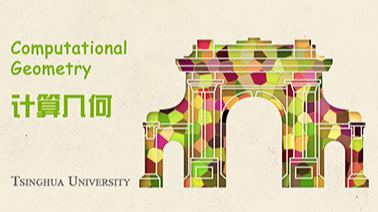# Computational Geometry

In this introductory computer science course, explore geometry, develop geometric thinking, and learn geometric algorithms.

## Computational Geometry课程简介：前往报名学习## Computational Geometry课程简介：

In this introductory computer science course, explore geometry, develop geometric thinking, and learn geometric algorithms.

No list

## Computational Geometry授课教师：

### 邓俊辉-教授-清华大学-计算机科学与技术系